Study Materials

# NCERT Solutions for Class 9th Mathematics

Page 8 of 9

## Chapter 13. Surface Areas and Volumes

### Exercise 13.8

EXERCISE 13.8

Assume π =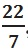, unless stated otherwise.

1. Find the volume of a sphere whose radius is

(i) 7 cm                            (ii) 0.63 m

Solution: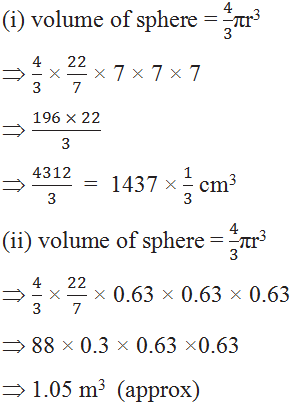2. Find the amount of water displaced by a solid spherical ball of diameter

(i) 28 cm                                     (ii) 0.21 m

Solution: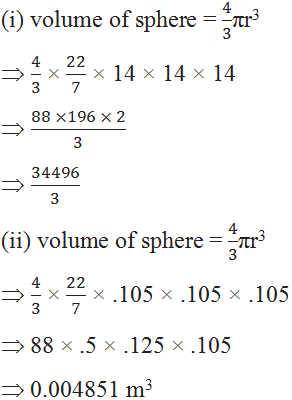3. The diameter of a metallic ball is 4.2 cm. What is the mass of the ball, if the density of the metal is 8.9 g per cm3 ?

Solution: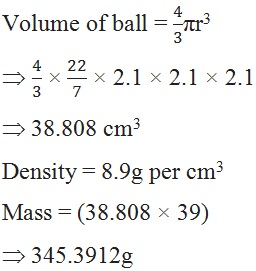4. The diameter of the moon is approximately one-fourth of the diameter of the earth. What fraction of the volume of the earth is the volume of the moon?

Solution:

Let diameter of earth = x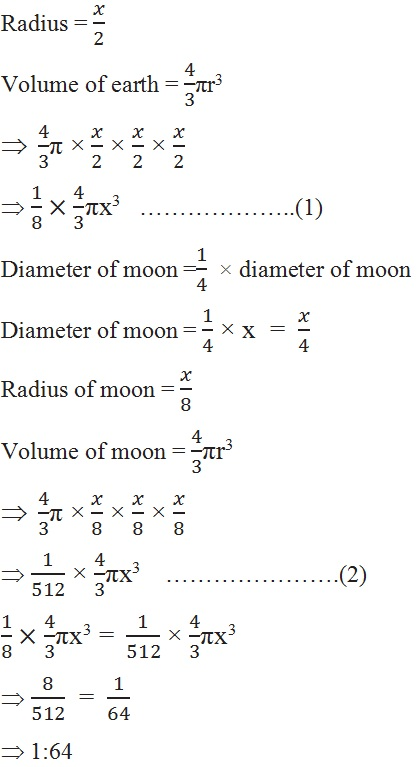5. How many litres of milk can a hemispherical bowl of diameter 10.5 cm hold?

Solution:

Diameter = 10.5 ,  radius = 5.25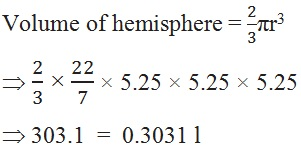6. A hemispherical tank is made up of an iron sheet 1 cm thick. If the inner radius is 1 m, then find the volume of the iron used to make the tank.

Solution: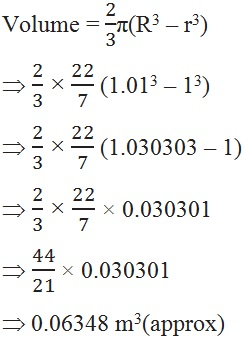7. Find the volume of a sphere whose surface area is 154 cm2.

Solution:

Surface area = 154 cm2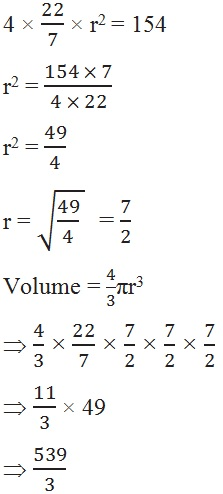8. A dome of a building is in the form of a hemisphere. From inside, it was white-washed at the cost of 498.96. If the cost of white-washing is 2.00 per square metre, find the

(i) inside surface area of the dome, (ii) volume of the air inside the dome.

Solution: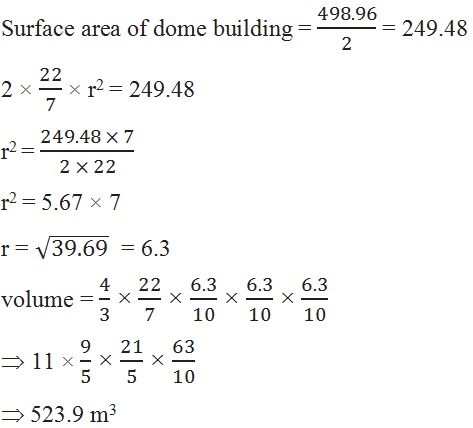9. Twenty seven solid iron spheres, each of radius r and surface area S are melted to form a sphere with surface area S2. Find the

(i) radius r2 of the new sphere,                  (ii) ratio of S and S2.

Solution:

R = r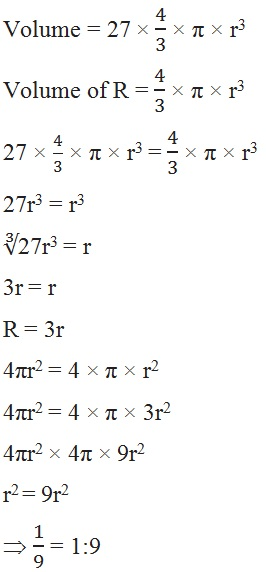10. A capsule of medicine is in the shape of a sphere of diameter 3.5 mm. How much medicine (in mm3) is needed to fill this capsule?

Solution :

Diameter = 3.5 mm ,  r = 1.75 mm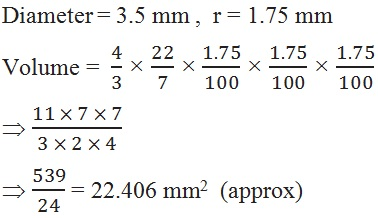Page 8 of 9

Chapter Contents: